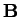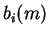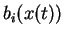Next: Learning algorithms Up: Hidden Markov models Previous: Hidden states   Contents

## Continuous observations

An obvious extension to the basic HMM model is to allow continuous observation space instead of a finite number of discrete symbols. In this model the parameterscannot be described as a simple matrix of point probabilities but rather as a complete pdf over the continuous observation space for each state. Therefore the values ofin Equation (4.4) must be replaced with a continuous probability distribution(4.7)

This model is called continuous density hidden Markov model (CDHMM). The probability of an observation sequence evaluated in Equation (4.5) stays the same. The conditional distributionscan in principle be arbitrary but usually they are restricted to be finite mixtures of simple parametric distributions, like Gaussians .

Antti Honkela 2001-05-30